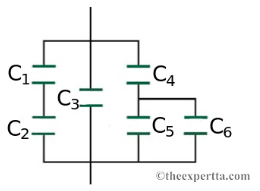# Problem: A combination of series and parallel connections of capacitors is shown in the figure. The sizes of these capacitors are given by the follow data:C1 = 4.1 μFC2 = 3.2 μFC3 = 7.7 μFC4 = 1.6 μFC5 = 0.65 μFC6 = 13 μF Find the total capacitance of the combination of capacitors in microfarads.

###### FREE Expert Solution

For 2 capacitors in series, the equivalent capacitance is:

$\overline{){{\mathbf{C}}}_{\mathbf{e}\mathbf{q}}{\mathbf{=}}\frac{{\mathbf{C}}_{\mathbf{1}}{\mathbf{C}}_{\mathbf{2}}}{{\mathbf{C}}_{\mathbf{1}}\mathbf{+}{\mathbf{C}}_{\mathbf{2}}}}$

For capacitors in parallel, the equivalent capacitance is:

$\overline{){{\mathbf{C}}}_{\mathbf{e}\mathbf{q}}{\mathbf{=}}{{\mathbf{C}}}_{{\mathbf{1}}}{\mathbf{+}}{{\mathbf{C}}}_{{\mathbf{2}}}{\mathbf{+}}{\mathbf{.}}{\mathbf{.}}{\mathbf{.}}{\mathbf{+}}{{\mathbf{C}}}_{{\mathbf{n}}}}$

Capacitor C5 and C6 are in parallel.

C56 = 0.65 + 13 = 13.65 μF

99% (178 ratings)###### Problem DetailsA combination of series and parallel connections of capacitors is shown in the figure. The sizes of these capacitors are given by the follow data:

C1 = 4.1 μF
C2 = 3.2 μF
C3 = 7.7 μF
C4 = 1.6 μF
C5 = 0.65 μF
C6 = 13 μF

Find the total capacitance of the combination of capacitors in microfarads.